Wednesday, September 30, 2009

Quick study

Yesterday I came across a very nice set of quick explanations of what Apple calls "Core competencies." It's very well done, highly recommended and particularly suited to the impatient.

Writer's block

In my early days with Cocoa (using PyObjC) one of the hardest things to wrap my head around was the byzantine nature of the way you ask the user for a filename to save to (or open). What can be difficult about that?

The function has one of the longest names I've ever seen:

- (void)beginSheetForDirectory:(NSString *)path file:(NSString *)name modalForWindow:(NSWindow *)docWindow modalDelegate:(id)modalDelegate didEndSelector:(SEL)didEndSelector contextInfo:(void *)contextInfo

The modal part isn't bad---it just means we'll stop everything else for the user (in that application anyway) until they please answer the <frickin'> question. The modalDelegate and contextInfo can be nil. Great!

The complication came with selectors (what are they?) and how do we get one in Python? Suddenly I had to learn about method signatures and other arcanery when all I wanted to do was save something...

Now, there's a new world: blocks.

The old method is deprecated (that's Cocoa for "it sucks to be you"). The new method defined in the docs is:

- (void)beginSheetModalForWindow:(NSWindow *)window completionHandler:(void (^)(NSInteger result))handler

It is shorter, but WTF? What is that caret-like thing sticking up there? What's with the double parens "))" and all the brackets? That's a block. It's like a Python lambda (section 4.7.5). Also known as a closure. And most informatively: an "anonymous function."

In my code, it looks something like this:

 [savePanel beginSheetModalForWindow:[NSApp mainWindow] completionHandler:^(NSInteger result) { if (result == NSOKButton) { [savePanel orderOut:self]; [self application:NSApp saveFile:[savePanel filename]]; } }];

What this:

(void (^)(NSInteger result))handler

says (in the Cocoa docs, first example) is the following. When the modal dialog is finished, there will be an integer result that describes which button the user clicked. We are going to pass into the modal dialog handling part of Cocoa a "completionHandler".

What is that? It is a function with no name that we are going to define on the spot. It doesn't return anything (and we can leave that out in our implementation), but it expects an NSInteger argument named result. The next five lines (including the first curly bracket) are the code for our function, followed by another curly bracket that terminates the block, and followed (finally) by a straight bracket that ends the message to the savePanel.

Sneakily, we call the method to actually save the file from within this anonymous function that takes a simple NSInteger and returns nothing.

That's the basic explanation. Blocks are apparently way more useful than that. But this, you have to know.

NSDate

I'm pretty sure this is not the official way to do it, but an easy way to extract the year-month-date information from an NSDate instance is to do

componentsSeparatedByString:@" "

on the description. Also, notice that constructing an NSDate object from a string requires a complete specification---including the time zone!

And at first I thought, there seems to be a bug in Apple's implementation of the dateWithString method. We specified January 1, but the resulting NSDate is one day (and one year) earlier. The answer lies in the time zone. Cocoa has converted my GMT time in constructing the NSDate to the current time zone where I am, even correcting for daylight savings time on the appropriate dates.

 today 2009-09-30 16:05:32 -0400array a ( "2009-09-30", "16:05:32", "-0400")array b ( 2009, 09, 30)birthday 2000-12-31 19:00:00 -0500present age (seconds) 276033932.002803age doubled 2018-06-30 12:11:04 -0400age halved 2005-05-17 06:02:46 -0400

 // gcc -o test test3.m -framework Foundation -fobjc-gc-only// ./test#import int main (int argc, const char * argv[]) { //NSCalendarDate is deprecated NSDate *today = [NSDate date]; NSLog(@"today %@", [today description]); NSArray *a = [[today description] componentsSeparatedByString:@" "]; NSArray *b = [[a objectAtIndex:0] componentsSeparatedByString:@"-"]; NSLog(@"array a %@", a); NSLog(@"array b %@", b); // creating a date from a string // requires all the pieces! NSString *s = @"2001-01-01 00:00:00 +0000"; NSDate *bd = [NSDate dateWithString:s]; NSLog(@"birthday %@", [bd description]); NSTimeInterval ti = [today timeIntervalSinceDate:bd]; // it is wrong to use d here! NSLog(@"present age (seconds) %f", ti); // OS X 10.6 only NSDate *ageDoubled = [NSDate dateWithTimeInterval:ti sinceDate:[NSDate date]]; NSLog(@"age doubled %@", ageDoubled); NSDate *ageHalved = [NSDate dateWithTimeInterval:(ti/2) sinceDate:bd]; NSLog(@"age halved %@", ageHalved); return 0;}

The number of the beast

As you may have noticed, I get a little bit obsessed. Right now, I'm working hard on Cocoa, next month it'll probably be something else. And I want to use the blog as a way to (in Bill Bumgarner's memorable phrase) "so Google can organize my head." This post is tagged "Instant Cocoa." The idea is to present a short, succinct exploration of some aspect of Cocoa with working code. Now, that code may break in the future, but it seems like a good idea right now.

And a disclaimer: these posts are for someone exactly like me. In fact, they are for me. If you know anything about Cocoa, you are probably wasting your time. But if you're struggling with the documentation and overly complex examples, you've come to the right place.

NSNumber

NSNumber provides a way of storing a value (the docs say: "a signed or unsigned char, short int, int, long int, long long int, float, or double or a BOOL." You can't do math with these things, but you can store them in an array and write them to disk, very useful indeed. You can also compare them, and get them back out again when you need them.

So I had a couple of questions:

• what 's the difference between the class method and instance method for getting a new number?

+ (NSNumber *)numberWithInteger:(NSInteger)value
- (id)initWithInteger:(NSInteger)value

• why is there a method for int and also a method for integer?

• I saw a discussion of something funny going on with NSNumber, and I thought I'd explore it. See below.

You can copy and paste the code listing into a text file. Save it and compile it from the command line as indicated. In the first part, we do alloc..init. That means in the classical memory environment (as here b/c we compiled without garbage collection), we own the NSNumber and must should release it when we're done with it.

Curiously, this freshly minted NSNumber has a retainCount of 2. I thought we owned it, but it looks like we we're just renting...

After that we get a second NSNumber using the same integer value (1) by the class "convenience" method. This code fails unless we set up the autorelease pool beforehand. Something very wild happens. It has a retainCount of 3, and remarkably, the retainCount of our first NSNumber has also gone up. Can you guess?

We really shouldn't, but let's do a retain on the first number in the second part of the code. Once again, the retainCount of both NSNumbers is incremented. What's going on? A peek at the addresses of the objects reveals the magic: they actually are the same thing! Here's the output (stripped of the date/time info from NSLog):

 n1=1 count=2n1=1 count=3 n2=1 count=3n1=1 count=4 n2=1 count=40x100108ce0 0x100108ce0break on 13

The last line of the output results from the third section of code. We go through the integers one-by-one until the NSNumbers we get from calls to the class method are different. This happens when i = 13. For 0 through 12 they are identical. It's a baker's dozen.

 // gcc -o test test4.m -framework Foundation// ./test// no garbage collection#import int main (int argc, const char * argv[]) { NSNumber *n1 = [[NSNumber alloc] initWithInteger:1]; NSLog(@"n1=%@ count=%u",n1,[n1 retainCount]); NSAutoreleasePool * pool = [[NSAutoreleasePool alloc] init]; NSNumber *n2 = [NSNumber numberWithInteger:1]; NSLog(@"n1=%@ count=%u n2=%@ count=%u", n1,[n1 retainCount],n2,[n2 retainCount]); [n1 retain]; NSLog(@"n1=%@ count=%u n2=%@ count=%u", n1,[n1 retainCount],n2,[n2 retainCount]); NSLog(@"%p %p",n1,n2); NSNumber *x; NSNumber *y; NSString *s; NSString *t; NSUInteger i; for (i=0; i<100; i++) { x = [NSNumber numberWithInteger:i]; y = [NSNumber numberWithInteger:i]; s = [NSString stringWithFormat:@"%p",x]; t = [NSString stringWithFormat:@"%p",y]; if (!([s isEqual:t])) { break; } } NSLog(@"break on %u", i); [pool drain]; return 0;}

[blogger is doing weird sh*t with the indentation on my code. Sorry about that. Let me know if something doesn't work for you.] And let me know if you have a better idea about code posting in html.

Tuesday, September 29, 2009

This post is the result from some more tests I've run on archiving. Some interesting points:

• I'm compiling and running the tests from the command line. I've enabled garbage collection as you can see in the compiler invocation on the first line of the listing.

• The object to be archived is an instance of NSColor. In order to use this class, I need to not only import the AppKit but also link against it. I missed that at first.

• Just for fun, I get a color from the "Crayons" color list.

• The last example used NSKeyedArchiver, but since we're not using a key, we can just use NSArchiver.

• The interesting part is where the action happens in:

 [archiver encodeObject:maroon];

Essentially, the archiver says to the object: encode yourself! Since NSColor conforms to NSCoding, it should (and fortunately, is) able to do that.• The encoded color is an NSMutableData object, and can now be added to an array and written to a plist file.Output:

 2009-09-29 16:13:04.573 test[1077:10b] color NSCalibratedRGBColorSpace 0.501961 0 0.25098 12009-09-29 16:13:04.576 test[1077:10b] data <040b7374 7265616d 74797065 6481e803 84014084 8484074e 53436f6c 6f720084 84084e53 4f626a65 63740085 84016301 84046666 66668381 80003f00 83818080 3e0186>2009-09-29 16:13:04.582 test[1077:10b] array1 ( <040b7374 7265616d 74797065 6481e803 84014084 8484074e 53436f6c 6f720084 84084e53 4f626a65 63740085 84016301 84046666 66668381 80003f00 83818080 3e0186>)2009-09-29 16:13:04.582 test[1077:10b] filename /Users/te/Desktop/a.plist2009-09-29 16:13:04.585 test[1077:10b] color NSCalibratedRGBColorSpace 0.501961 0 0.25098 1

Code listing:

 // gcc -o test test2.m -framework Foundation -framework AppKit -fobjc-gc-only// ./test#import #import int main (int argc, const char * argv[]) { NSColor *maroon; NSColorList *CL; CL = [NSColorList colorListNamed:@"Crayons"]; maroon = [CL colorWithKey:@"Maroon"]; NSLog(@"color %@", maroon); NSMutableData *mdata; mdata = [[NSMutableData alloc] initWithCapacity:100]; NSArchiver *archiver; archiver = [[NSArchiver alloc] initForWritingWithMutableData:mdata]; [archiver encodeObject:maroon]; NSLog(@"data %@", [mdata description]); NSArray *arr; arr = [NSArray arrayWithObject:mdata]; NSLog(@"array1 %@", arr); NSString *fn; bool result; fn = NSHomeDirectory(); fn = [fn stringByAppendingString: @"/Desktop/a.plist"]; NSLog(@"filename %@", fn); [arr writeToFile:fn atomically:YES]; NSArray *arr2; arr2 = [NSArray arrayWithContentsOfFile:fn]; NSColor *color; NSData *data = [arr2 objectAtIndex:0]; color = [NSUnarchiver unarchiveObjectWithData:data]; NSLog(@"color %@", color); return 0;}

Cocoa: NSKeyedArchiver

For a Cocoa application, I want to save an NSColor in a plist file on disk. Saving and loading data like strings and NSNumbers from such a file is easy. Just use writeToFile: atomically (or writeToURL: atomically:), and reload the data with arrayWithContentsOfURL:

 - (void)awakeFromNib { NSMutableArray *mA = [NSMutableArray arrayWithObjects:@"a",@"b",nil]; NSString *fn = NSHomeDirectory(); fn = [fn stringByAppendingString:@"/Desktop/xyz.plist"]; NSURL *fileURL = [NSURL fileURLWithPath:fn]; [mA writeToURL:fileURL atomically:YES]; NSMutableArray *mA2 = [NSMutableArray arrayWithContentsOfURL:fileURL]; NSLog(@"%@", mA2);}

The Console logs the output (some extra stuff has been stripped out):

 .. ( a, b)

However, if you try the same procedure with an array containing things that can't be saved in this way (like NSColor) the file write method fails silently, with no indication of why it failed. You must do something like this:

 - (void)awakeFromNib { NSMutableArray *mA = [NSMutableArray arrayWithObjects:@"a",@"b",nil]; NSColor *c = [NSColor blueColor]; [mA addObject:c]; int i; for (i = 0; i < [mA count]; i++) { id obj = [mA objectAtIndex:i]; if ([[obj class] isEqual:[c class]]) { NSLog(@"%@", obj); id obj2 = [NSKeyedArchiver archivedDataWithRootObject:c]; [mA replaceObjectAtIndex:i withObject:obj2]; } } NSString *fn = NSHomeDirectory(); fn = [fn stringByAppendingString:@"/Desktop/xyz.plist"]; NSURL *fileURL = [NSURL fileURLWithPath:fn]; [mA writeToURL:fileURL atomically:YES]; NSMutableArray *mA2 = [NSMutableArray arrayWithContentsOfURL:fileURL]; id obj = [mA2 objectAtIndex:2]; NSColor *c2 = [NSKeyedUnarchiver unarchiveObjectWithData:obj]; [mA2 replaceObjectAtIndex:2 withObject:c2]; NSLog(@"%@", mA2);}

 .. NSCalibratedRGBColorSpace 0 0 1 1.. ( a, b, "NSCalibratedRGBColorSpace 0 0 1 1")

The actual archiving step is a single line. In this case, however, the array is a mixture of different types. For the archiving step, I can test "if ([[obj class] isEqual:[c class]])", but on unarchiving, I think I have to know which objects need to be unarchived. Cocoa knows what they are, but I don't.

There is probably a better way to keep it all straight. Tell me if you know.

Saturday, September 26, 2009

Cocoa: TableView --- one more thing

Continuing the last post, there is one more thing.

On the question of how to change the Table View programmatically, I finally read the relevant section of the docs, which is titled:

Programmatically Modifying a Controller’s Contents

Make the array controller an outlet, then respond to the add button by modifying it like so:

 NSInteger i; i = [TVController selectionIndex]; if (i == NSNotFound ) { i = [data count]; } [TVController insertObject:mD atArrangedObjectIndex:i]; NSLog(@"add %@ %i", [data description], i);

The change will propagate back to the data source automatically. Note that you will have to do a little more fiddling to deal with a multiple selection, or just disable it.

Cocoa: TableView bindings---simplest possible example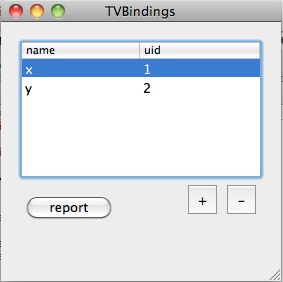This is without doubt the world's simplest NSTableView bindings example. To try it out, create a standard XCode Cocoa application and add the relevant items from this post to it.

The AppDelegate has an init method, which calls another function to load some preliminary data from a plist file. This has to happen before "awakeFromNib" or the TV won't display anything when it launches. I put the data in a plist file in resources for convenience, but you could easily construct it from scratch. I show the scratch version because the plist won't display properly in html:

 - (id)init { self = [super init]; if (self == nil) return nil; [self loadArray]; return self;} - (void)loadArray{ data = [NSMutableArray arrayWithCapacity:10]; NSMutableDictionary *mD = [ NSMutableDictionary dictionaryWithCapacity:2]; [mD setObject:@"x" forKey:@"name"]; [mD setObject:@"1" forKey:@"uid"]; [data addObject:mD]; NSMutableDictionary *mD2 = [ NSMutableDictionary dictionaryWithCapacity:2]; [mD2 setObject:@"y" forKey:@"name"]; [mD2 setObject:@"2" forKey:@"uid"]; [data addObject:mD2]; [data retain];}

If you wanted to load the data from a plist resource, you would do something like this instead:

 - (void)loadArray{ NSString *fn = [NSString stringWithString:@"data"]; NSString *path = [[NSBundle mainBundle] pathForResource:fn ofType:@"plist"]; data = [NSMutableArray arrayWithContentsOfFile:path]; [data retain];}

The data variable is declared in the header as usual (no getters or setters or @property stuff):

 #import @interface TVBindingsAppDelegate : NSObject { NSWindow *window; NSMutableArray *data;}@property (assign) IBOutlet NSWindow *window;- (void)loadArray;- (IBAction)report:(id)sender;@end

The AppDelegate also has a method so I can see what has happened to the data source.

 - (IBAction)report:(id)sender{ NSLog(@"data %@", [data description]);}

That's all the code there is! The window of the running application, again:In IB, drag an array controller onto the nib:Here are the bindings. Be sure the table columns are selected when you bind to the array controller.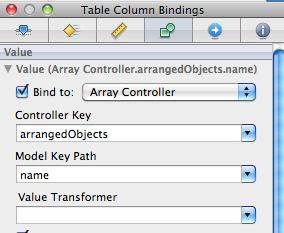As shown in the screenshot, there is a Table View with two columns. Editing works, and the + and - buttons add new rows or delete the selected row. Connect the + and - buttons by control drag to the controller, and report to the App Delegate, as usual. Check the connections for the array controller.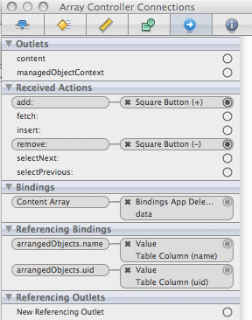Make sure the array controller knows the right class to add to the array: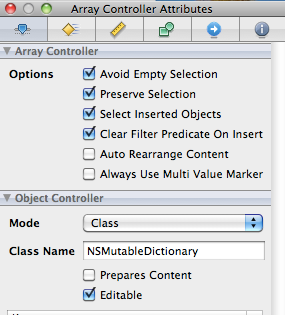One thing I don't know how to do yet is to change the default behavior, which only adds at the end, to add a new row after the selected row, say, at the second position. I suppose the way to do that is to connect the buttons to the App Delegate and modify the data source.

Thursday, September 24, 2009

Cocoa: sorting an array

I went poking around in the header file "NSArray.h" and found some methods that were new to me.

• firstObjectCommonWithArray:
• removeObject:
• exchangeObjectAtIndex: withObjectAtIndex:
• sortedArrayUsingDescriptors:

 // gcc -o arrays arraystuff.m -framework Foundation// ./arrays#import int main (int argc, const char * argv[]) { NSAutoreleasePool * pool = [[NSAutoreleasePool alloc] init]; NSUInteger i; NSMutableArray *mA1 = [NSMutableArray arrayWithCapacity:10]; NSMutableArray *mA2 = [NSMutableArray arrayWithCapacity:10]; for (i = 0; i < 6; i++) { [mA1 addObject:[NSNumber numberWithInt:i]]; } for (i = 5; i < 10; i++) { [mA2 addObject:[NSNumber numberWithInt:i]]; } NSNumber *x = [mA1 firstObjectCommonWithArray:mA2]; NSLog(@"x %@", [x description]); [mA2 removeObject:[NSNumber numberWithInt:6]]; NSLog(@"mA2 %@", [mA2 description]); [mA1 exchangeObjectAtIndex:0 withObjectAtIndex:2]; NSLog(@"mA1 %@", [mA1 description]); NSSortDescriptor *sd; sd = [[[NSSortDescriptor alloc] initWithKey:@"intValue" ascending:NO] autorelease]; NSArray *sdA = [NSArray arrayWithObject:sd]; NSArray *a1 = [mA1 sortedArrayUsingDescriptors:sdA]; NSLog(@"a1 %@", [a1 description]); [pool drain]; return 0;}

 .. \$ ./arrays.. x 5.. mA2 ( 5, 7, 8, 9).. mA1 ( 2, 1, 0, 3, 4, 5).. a1 ( 5, 4, 3, 2, 1, 0)

Cocoa: strip for NSString

A few weeks ago I was complaining that NSString doesn't have a strip method like I use all the time in Python. I was wrong. It's called stringByTrimmingCharactersInSet:. Here is a bit of code (run from the command line) that exercises three useful NSString functions:

• componentsSeparatedByString
• componentsSeparatedByCharactersInSet
• stringByTrimmingCharactersInSet

 // gcc -o strings stringstuff.m -framework Foundation// ./strings#import int main (int argc, const char * argv[]) { NSAutoreleasePool * pool = [[NSAutoreleasePool alloc] init]; NSString *s = [[NSString alloc] initWithString:@"v\nx y;z"]; NSArray *a = [s componentsSeparatedByString:@"\n"]; NSLog(@"a = %@", [a description]); NSCharacterSet *cs = [NSCharacterSet characterSetWithCharactersInString:@"\n ;"]; a = [s componentsSeparatedByCharactersInSet:cs]; NSLog(@"a = %@", [a description]); NSString *s2 = [[NSString alloc] initWithString:@" x y z "]; cs = [NSCharacterSet characterSetWithCharactersInString:@" "]; NSString *s3 = [s2 stringByTrimmingCharactersInSet:cs]; NSLog(@"s3 = %@, length %u", [s3 description], [s3 length]); [s release]; [s2 release]; [pool drain]; return 0;}

Here is the output:

 .. \$ ./strings.. s = vx y;z.. a = ( v, "x y;z").. a = ( v, x, y, z).. s3 = x y z, length 5

Cocoa: command line arguments

I found myself trying to write a "Foundation tool" that would process a command line argument. It's not really clear to me why I wouldn't use Python to do this, but that's another story. So I wrote the following code in a file "CL1.m" on my Desktop:

 #import int main (int argc, const char * argv[]) { NSAutoreleasePool * pool = [[NSAutoreleasePool alloc] init]; if (argc > 1) { printf("printf %s\n",argv); NSData *d = [NSData dataWithBytes:argv length:4]; NSLog(@"data: %@",[d description]); NSString *s = [[NSString alloc] initWithCString:argv encoding:NSASCIIStringEncoding]; NSLog(@"string description: %@",[s description]); // this doesn't work! NSLog(@"string: %s", s); } [pool drain]; return 0;}

Compile like this:

 gcc -o CL1 CL1.m -framework Foundation

and run from the command line:

 .. \$ ./CL1 xyz printf xyz.. data: <78797a00>.. string description: xyz.. string: †¥u†å

I looked at the data directly, because I was (wrongly) using NSLog as shown in the last call, and I wanted to look at the bytes directly. As expected, hex 78 is decimal 7*16 + 8 = 120, which is 'x' in ascii.

However, there is a better way to do this, as discussed in this post. NSUserDefaults will read command line arguments. It works like so:

 #import int main (int argc, const char * argv[]) { NSAutoreleasePool * pool = [[NSAutoreleasePool alloc] init]; NSUserDefaults *args = [NSUserDefaults standardUserDefaults]; NSLog(@"arg = %@ ", [args stringForKey:@"input"]); [pool drain]; return 0;}

 .. \$ ./CL2 -input xyz.. arg = xyz

As discussed in the post, this method is really versatile, it can parse for integers or floats or booleans. (If you try to copy and paste this code, be sure to fix the < symbol in the import statement).

You can get an idea what is in the dictionary by doing this from the command line:

Monday, September 21, 2009

More on parabolas

Let's continue with the previous problem. We have the parabola
 y = x2y' = 2x

The slope at any point is 2x. To find the focus, we require the y-value where the slope equals 1, that occurs when
 x = 1/2, y = 1/4

So the focus is at the point:
 x = 0, y = 1/4

Now, we want to consider all points x. At every such point
 y = x2

The line from the focus (superimposed in magenta on our old diagram) has the slope at every point of:

 (x2 - 1/4) / xRather than deal explicitly with calculating angles, I'm going to use vectors. There are three of them in this problem. It doesn't matter whether we take the vectors in their forward or reverse directions, so I'll consider IN as

the reverse of the unit vector of light coming in:

 IN = [0,1]

The reverse of the vector of light coming out calculated above, and the slope are:

 OUT = [x, x2 - 1/4]M = [1, 2x]

What we are trying to prove is that the angle between OUT (magenta arrow) and M (slope or tangent to the curve at the magenta point) is the same as the angle between IN (blue dotted line) and M.

In vector algebra, the dot product

 A • B = Σ Ax Bx + Ay By = |A| |B| cos θ

where the absolute value of the vectors comes from Pythagoras, and θ is the angle between them. In the language of vectors, we claim that the angles for the two vector comparisons are the same, and therefore:

 IN • M / |IN| |M| = OUT • M / |OUT| |M|IN • M / |IN| = OUT • M / |OUT|IN • M = 0 * 1 + 1 * 2x = 2x|IN| = 1OUT • M = x + 2x * (x2 - 1/4)|OUT| = √ [x2 + (x2 - 1/4)2 ]

So the left-hand side is just 2x.

The right-hand side looks complicated but it is easily simplified. The numerator is:

 x + 2x * (x2 - 1/4) = 2x (1/2 + x2 - 1/4) = 2x (x2 + 1/4)

The denominator is:

 √ [x2 + (x2 - 1/4)2 ] = √ [x2 + x4 -1/2 x2 + 1/16 ] = √ [x4 + 1/2 x2 + 1/16 ] = √ [(x2 + 1/4)2 ] = x2 + 1/4

Cancel x2 + 1/4 from both top and bottom, leaving 2x, and the identity is verified! There is one more question, however, and that is whether we can generalize this result to any parabola. That will have to wait.

Focus on parabolas

In Strang I read that a parabola has a point called the focus.

Imagine that light rays are coming from the stars down a telescope to its reflective mirror, that is, vertically down to the inside surface of a parabola. All such rays are supposed to be reflected to the same point, which is the focus.

For one particular (positive) x, the vertical rays will be reflected along a horizontal line.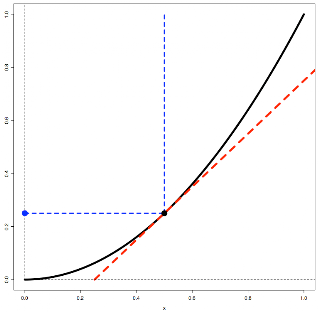In the figure, I plotted a part of the parabola y = x2, and also its tangent line at the point (0.5,0.25) which has a slope of 1 (2 times x).

The solution for this particular point is easy. According to the law of reflection, the angle between the vertical blue line of the incoming ray and the slope line (red) is equal to the angle between the outgoing horizontal blue line and the slope. This happens when the red line has a slope of 1 and the angle (call it θ) is π/4.

At any other point (except x = - 0.5), the angle the vertical line makes with the slope is not π/4 and the outgoing ray is not horizontal. But the claim is that the outgoing ray still passes through the point (0,0.25).

I'm going to see if I can prove that. I'll report back later.

R code:
 f <- function(x) { x**2 }plot(f,from=0,to=1, col='black',lwd=5)m <- function(x) { x - 0.25}plot(m,from=0.25,to=1.5, col='red',lty=2,lwd=5,add=T)lines(c(0,0.5),c(0.25,0.25),lty=2, lwd=3,col='blue')lines(c(0.5,0.5),c(0.25,1),lty=2, lwd=3,col='blue')points(0.5,0.25,col='black',pch=16,cex=3)points(0,0.25,col='blue',pch=16,cex=3)lines(c(0,0),c(0,4),lty=2)lines(c(0,2),c(0,0),lty=2)

Tuesday, September 15, 2009

Calculus in 5 minutes (last part)I am unable to resist saying a word or two about how we get the standard method. I can't emphasize enough that you don't need to know this to use calculus! But it might be interesting. Here goes...

Remember:

 y = xny' = n xn-1

Where does this come from?

Well, what is the slope of the curve at any point x? Imagine that we increase x by a small amount, we'll call that amount h. The slope of the curve is the difference in y [ the value of the function f(x) ], between the two points x and x + h. How much does it change?

Suppose:

 y = x3slope = [ f(x + h) - f(x) ] / hf(x + h) = (x + h)3 = ?

Well,

 (a + b)n is the binomial expansion of a + b(a + b)n = an + n an-1 b + stuff times b2 + more stuff

So...

 (x + h)n = xn + n xn-1 h + stuff times h2 + more stuff

And there are three quick points:

• (1) The first term xn is what we need to subtract, it is f(x)

• (2) The third and following terms involve h2 (and higher powers). We ignore these terms!

 Suppose h = 10-6, then h2 = 10-12 Not small enough? Suppose h = 10-60, then h2 = 10-120 Not small enough?…

The point is that h2 goes away faster than h as h gets very small, and if you don't think it's getting small fast enough, just choose h to be even smaller...

• (3) What remains is the first term of the binomial expansion:

 n xn-1 h

To get the slope, we need to divide by h:

 slope = n xn-1 h / h

The h / h part is still equal to 1 even when h gets very small. So it goes away!

 slope = n xn-1

There you have it.

As I said, this is for my son. Here is a picture from an earlier time:Calculus in 5 minutes (part 4)

If you haven't read the earlier posts, they are here, here and here.

Now that was interesting, you say. But how about a little proof ? For a mathematician, the word "proof" is like waving the red handkerchief to a bull at Pamplona, but I'm not going there. However, it is always a good idea to check things a bit to see if they make sense. Consider the simple equation:

 y = x2

We want to know the area under the curve.I know how to do that, you say. Remembering that the curve itself is the derivative of the area function f(x), we have:

 y' = x2y = 1/3 x3evaluated between x = 1 and x = 0:area = 1/3

That was way too easy. But consider this: how about the area above the curve?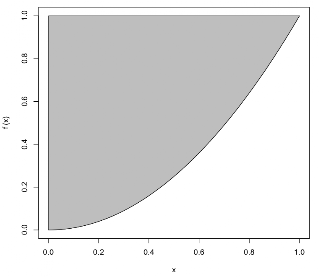Notice that we have

 y = x2x = √y = y1/2

I get a little freaked out seeing x as a function of y, so I am going to mentally turn the curve through 90 degrees and then make its mirror image reflection. I get this:Now, our differentiation trick works for fractional exponents. So we have:

 y' = x1/2y = 2/3 x3/2evaluated between x = 1 and x = 0:area = 2/3

And, as we might hope and expect, 2/3 + 1/3 does equal the area of the unit square. And note that it will work for any power of x. If, for example, we start with the curve y = x3, then the first area is 1/4 x4, and the second area is 3/4 x4/3. It's easy to see that it works for any rational power of x. Suppose we have:

 y' = xa/bthe exponent is a/b, leta/b + 1 = c/by = b/c xc/bx' = yb/ab/a + 1 = d/ax = a/d yd/aFrom above:a/b + 1 = c/ba + b = cAlso from above:b/a + 1 = d/aa + b = dSo:c = da + b = ca/c + b/c = 1a/d + b/c = 1

As we guessed, we see that b/c + a/d = 1.

(Of course, it helps that we chose the upper limit for measuring the area at x = 1, y = 1. Evaluation for some other bound would involve a bit more calculation).

Ladies and gentlemen, will you please…give it up for Mr. Leibnitz and Mr. Newton.

[Update: blogger messed with my code. Think I fixed it:]

R code:
 f <- function(x) { return (x**2) } plot (f,0,1,cex=3) xvals = seq(0,1,length=1000) yvals = f(xvals) x = c(xvals,rev(xvals)) y = c(rep(0,1000),rev(yvals)) polygon(x,y,col='gray')f <- function(x) { return (x**2) } plot (f,0,1,cex=3) xvals = seq(0,1,length=1000) yvals = f(xvals) x = c(xvals,rev(xvals)) y = c(rep(1,1000),rev(yvals)) polygon(x,y,col='gray')f <- function(x) { return (x**0.5) } plot (f,0,1,cex=3) xvals = seq(0,1,length=1000) yvals = f(xvals) x = c(xvals,rev(xvals)) y = c(rep(0,1000),rev(yvals)) polygon(x,y,col='gray')

Calculus in 5 minutes (part 3)

This is a series about the essence, the merest whiff of the perfume of calculus. The first and second posts are here and here. Differentiation is useful, but integration allows us to do things which are nothing short of miraculous. For example, consider this modestly complex equation, and its curve.

 y = x3 - x2 - x + 150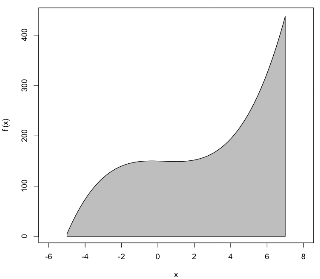The integral calculus (the second part) allows us to easily calculate the area under this curve (between the curve and the x-axis), as shown in gray.

Remember what we said before: the relationship between the function which we plot as a curve, and its slope at any point is found by differentiating f(x) to f '(x). The slope is f '(x). The secret of integral calculus (don't tell anybody) is that the same relationship holds between the area under a curve, and the curve itself, but in reverse. That is, if the curve is f '(x), the area is f(x). It's that simple.

If we know f '(x), how do we find f(x)? Sometimes it's easy and sometimes it's hard. Suppose that every time we have a function f(x) and we find f '(x), we write the result down and save it in our little black book. When we encounter a function in an integration problem, we look in the book.

For the example, we need a function f(x), which when differentiated gives:

 y' = x3 - x2 - x + 150

We look in our book, and there it is:

 y = x4/4 - x3/3 - x2/2 + 150 x

Of course, the area depends on which endpoints we choose. (Looking at the figure should make this clear). So evaluate the integrated function between the limits x = 7 and x = -5. We have:

 74/4 - 73/3 - 72/2 + 7*150minus(-5)4/4 - (-5)3/3 - (-5)2/2 + (-5)*150

I get:

 2401/4 - 343/3 - 49/2 + 1050 - 625/4 + 125/3 - 25/2 - 750

I'm too lazy to finish it. But I'll check the method itself by an interesting approach in the next post.

Why do I say it's a miracle? Because we get the area from the integrated equation just by using the endpoints. We don't have to worry about what lies between. That is amazing.

 R code:f <- function(x) { return (x**3 - x**2 - x + 150) } plot (f,-5,7,xlim=c(-6,8),cex=3) xvals = seq(-5,7,length=1000)yvals = f(xvals)x = c(xvals,rev(xvals))y = c(rep(0,1000),rev(yvals))polygon(x,y,col='gray')

Calculus in 5 minutes (part 2)In the first post of this series I mentioned that a car's odometer and speedometer perform related functions :), and these functions are a good way to think about the fundamental operations of calculus. Suppose a car is going in a straight line (say, on the Bonneville salt flats). We need to deal only with the positive x-axis.

Distance (often labeled s) is a function of time:

 s = f(t)

If the velocity is constant and we start from s = 0 then:

 s = v * t

Distance equals velocity times time. (60 miles per hour for 2 hours = 120 miles).

Now, velocity might be changing, it might be a function of time. Let's say we'll figure out how it changes later, and just write in a general way:

 v = f(t)

If the velocity is changing, we call the rate of change in velocity the acceleration. (It is the slope of the curve for v as a function of t). If the acceleration is constant (like gravity), then:

 v = a * t

For a car, imagine increasing the pressure on the gas pedal steadily so that after 1 second you are going 10 mph, after 2 seconds 20 mph, after 3 seconds 30 mph. If we continue at the same rate, we'll accelerate from 0 to 60 mph in 6 seconds.

As I said before, there is a special trick symbolized with a prime mark placed next to the s (the physicists put a dot on top). The trick is called differentiation:

 s' = ... something.

What it is depends on the exact form of s as a function of time. If the acceleration is constant:

 s = 1/2 a t2

Do you remember the rule from last time?

 y = c xny' = n c xn-1

Or, using the time and distance symbols:

 s = 1/2 a t2s' = v = a ts'' = a

So that is where the factor of 1/2 comes from in the equation involving acceleration. It is needed to cancel the the 2 that comes out of the exponent when we differentiate. One way to think about this is to say that the velocity after a period of constant acceleration is the average of the initial acceleration and the final acceleration, times the time. But this differentiation mumbo jumbo will work even if the velocity and acceleration are more complicated functions of time.

Sunday, September 13, 2009

Toolbars in Interface BuilderMaking a toolbar for a Cocoa application has become as simple as 1-2-3, with the use of Interface Builder. You just:

• drag a toolbar onto your window
• double click it to show "Allowed Toolbar Items"
• drag an "Image Toolbar Item" to the "Allowed Toolbar Items"
• set its Image Name to one present in your project resources
• drag the item from the "Allowed Toolbar Items" to the toolbar (not obvious at first)
• connect it to an IBAction in your controller

I have to confess I fooled around for a while because I didn't find the right Apple docs for this. Once you know how, it is truly painless.

Thursday, September 10, 2009

Slope

Here is a post I wrote for my son. I hope he reads it! The essential ideas of calculus are surprisingly simple. See if you don't agree.

We can talk about the slope of a curve at a single point. This seems odd because "slope" refers to a change in y divided by a change in x. We ought to need two points, not just one. But we don't.

A single point can have a slope for the same reason that the earth seems to be flat where I'm standing right now even though I know it is curved---it is flat, on the ocean or the Mohave desert anyway. The carpenters who built your house assumed that the earth's curvature is negligible, and we will too. We can mentally zoom in indefinitely (ask an ant if the earth is flat).So for any given point on a curve, we can always find another point very close nearby which has the same slope, and then we know the slope between them is constant (within any limits you want to establish).

Like driving a car

You can understand the basic procedures in calculus by thinking about driving a car (Strang). Most drivers refer frequently to the speedometer (measures velocity), if they're measuring distance they refer to the odometer.

If we plot accumulated distance as a function of time, the slope of that curve is the velocity shown on the speedometer. [This analogy will become useful in part 2].

Simple tricks

There are tricks to use to find the slope from the equation for a curve. Where the tricks come from isn't hard to understand, but it's not necessary---just use the trick. Consider the equation:

 (y-1) = (x-2)2

Multiply it out to make things a bit more interesting:

 y = x2 - 4x + 5The trick is that the slope of the curve (designated as y') is easily calculated:

 y = x2 - 4x + 5y' = 2x - 4

We used three rules together:

(i) if we have various parts being added together, we take the slope for each part separately and then add them up.

(ii) the slope of a constant term (like y = 3) is zero (obviously, a horizontal line is flat, and has slope = 0).

(iii) if (c stands for constant):

 y = c xn; y' = c n xn-1

So what

One reason this is useful is to find maximum and minimum points of a curve. These are points at which the slope is zero. For our example

 y' = 2x - 4 0 = 2x - 4 x = 2

This happens when

 y = x2 - 4x + 5y = 4 - 8 + 5 = 1

This is easy to see by going back to the first form of the equation. The minimum is when the term in parentheses on the right is zero.

 (y-1) = (x-2)2

There are other simple tricks for terms like 2x and sin x.

R code:
 f <- function(x) { x**2 - 4*x + 5}plot(f,from=-3, to = 7)plot(f,from=-3, to = 7,col='red',lwd=3)lines(c(-3,7),c(1,1),lty=2)lines(c(2,2),c(0,25),lty=2)

Tuesday, September 8, 2009

Heat Mapper rises from the ashes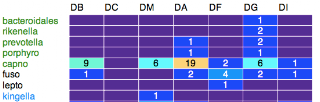This is the second of two posts about Apple-specific programming issues, so feel free to move on if you don't care about both subjects. I spent the labor day weekend re-doing my program to draw heat maps in Objective-C, and finally have a minimally functional version.

As usual, most of the time was wasted running down stupid errors, but I seem to be getting a little better about recognizing them. One of these had to do with a call like:

 IBOutlet id myController;

in a header file. This only works if said file is in the "Classes" section of Xcode. If it's in "Resources," as happened once by mistake, there is an error message from the compiler because the IB cannot actually connect to the outlet.

But the biggest time-waster (and probably the silliest bug) was caused by using spaces (long ago) to pad the the row names in the sample data which I feed to the application. It makes the text version look prettier (tabs don't always line up), as you can see in this sample without ljust:

 DB DC DM DAbacteroidales 0 0 0 0rikenella 0 0 0 0prevotella 0 0 0 1porphyro 0 0 0 1capno 9 0 6 19fuso 1 0 0 2lepto 0 0 0 0kingella 0 0 1 0neisseria 1 0 4 2

The problem came when I used a dictionary with genus names as keys to specify colors to draw the strings. The names I read from the data file were padded out to the length of the longest one:

 "bacteroidales""rikenella "

So the key lookup failed when searching for the second key but not for the first. It kinda worked… I spent at least 10 hours on this. Since writing code is laborious in Objective-C, writing testing code is also laborious.

On the other hand, the bug is solved, and if it ever comes again I hope to recognize the issue. Of course, if NSString had a strip method I would have used it it automatically as I do in Python, and it never would have happened.

Friday, September 4, 2009

Goodbye to all that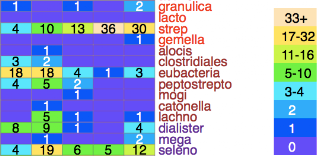This post is about Apple-specific programming issues, so feel free to move on if you don't care about both subjects. Perhaps the title is premature, and it is certainly melodramatic. But my recent upgrade to Snow Leopard broke my programs written using the PyObjC bridge (like HeatMapper), and what it looks like to me is that PyObjC is headed for the ash heap of history.

That pisses me off. The advantage of doing what I have been doing is to get a shiny GUI almost for free. Now comes the hurt, everything has to be redone, no matter what. If I have to learn get good at Objective C and integrate my Python parts with the ObjC parts, it's not such a good deal any more. Going the SVG route makes much more sense.

What happened: the app failed to launch, but that was no big surprise. Upgrade and s**t happens. So I go to XCode (the latest, v 3.2), and try to re-compile the project.

 ..._bridgesupport.py", line 53, in _parseBridgeSupport ))ValueError: Unknown typestr...

This happens when I do "import Foundation", which works, by the way, from the command line. I am suspicious, because Snow Leopard is not such a simple upgrade. 64-bit is wild. (Read Ars Technica).

But that's fine, I say, I can just fix what's wrong. So first I need a working PyObjC application. I go to create a new Project, but it turns out the templates for PyObjC stuff are no longer included in XCode.

You'll need to download and install the templates from the PyObjC repository or web site.

The templates were pulled from the release because the template development moves at a different pace & schedule than the Xcode releases. Too often, the templates have been out of date by the time the discs were pressed.

b.bum

 svn checkout http://svn.red-bean.com/pyobjc/trunk/pyobjc/pyobjc-xcode/Project%20Templates/

Follow the instructions in the readme...Look in XCode: there is a new thingie called User Templates. Make a new project using the template...it dies with the same error.

So I google: "leopard ValueError: Unknown typestr" and I find this:

As the maintainer of PyObjC for nearly 15 years, I will say it bluntly. Use Objective-C. You will need to know Objective-C to really understand Cocoa anyway and PyObjC is just going to add a layer of bugs & issues that are alien to 99% of Cocoa programmers.

– bbum 2 days ago

Can't get any more blunt than that. And I find this:
To be blunt:

If you want to be an effective Cocoa programmer, you must learn Objective-C. End of story.

Neither Python or Ruby are a substitute for Objective-C via their respective bridges. You still have to understand the Objective-C APIs, the behaviors inherent to NSObject derived classes, and many other details of Cocoa.

PyObjC and RubyCocoa are a great way to access Python or Ruby functionality from a Cocoa application, including building a Cocoa application mostly -- if not entirely -- in Python or Ruby. But success therein is founded upon a thorough understanding of Cocoa and the Objective-C APIs it is composed of.

b.bum
It is early days yet for Snow Leopard. Maybe it'll get fixed. You have to understand: Bill Bumgarner is the man. Well, him and Ronald Oussoren.

So what it looks like is that:

• Python is great on the Mac---from the command line
• PyObjC was fun, and great for the amateur programmer
• but, it was basically a complicated hack (thanks Bill and Ronald)
• I should follow bbum's advice and learn Objective C (it'll take time)
• you cannot depend on PyObjC to get you to Cocoa
• in the meanwhile, I am going to make my graphics with SVG

One bonus of this, of course, is that it'll be useful for people with PCs. But I think it's a sad day. Apple should think more about people who play on their computers but are not (smart enough or don't have the time to be) experts.

Thursday, September 3, 2009

More on the three-fold wayA while ago I posted about what seems to be a simple calculus problem---to find the area of a circle by integration. In fact we looked at three ways, and two were easy. But the first way is harder, and that involves y as a function of x with the goal to integrate y(x) from x = -R to x = R. At the time, I solved the problem by numerical integration.

The function I could not integrate is

 y = √(R2 - x2)

or sticking with a unit circle:

 y = √(1 - x2)

Reading further in Strang's Calculus, I find that there is a way to do it. The method has two parts: (i) a trigonometric substitution and (ii) integration by parts, which is a reversal of the product rule for differentiation.

Integration by parts

If we have two functions of x, u and v, and we want d/dx of uv, by the product rule we get:

 d/dx uv = u dv/dx + v du/dx

Thinking about integrating this (without the x's):

 uv = ∫u dv + ∫v du∫u dv = uv - ∫v du

So the trick is, when given a difficult integral, to try to imagine it transformed into ∫u dv. If ∫v du is easy or just easier, we have helped ourselves. Suppose we have:

 ∫cos2 x dx∫cos x cos x dx

Then let

 u = cos xdv = cos x dxv = sin xdu = -sin x dx∫cos2 x dx = = ∫u dv = uv - ∫v du = sin x cos x + ∫sin x sin x dx

And a trick:

 ∫cos2 x dx = = sin x cos x + ∫(1-cos2 x) dx = sin x cos x + x - ∫cos2 x dx

And another trick!:

 2 ∫cos2 x dx = sin x cos x + x∫cos2 x dx = 1/2(sin x cos x + x)

Amazing. And:

 ∫sin2 x dx = = ∫ (1 - cos2 x) dx = x - 1/2(sin x cos x + x) = 1/2(x - sin x cos x)

The "double angle" method

I quoted Strang the other day that:

 sin(s + t) = sin s cos t + cos s sin tcos(s + t) = cos s cos t - sin s sin t

These formulas lead to a pretty straightforward derivation of the integral of
cos2 t (happily, we get the same result as above):

if s = t then we have:

 sin 2t = 2 sin t cos tcos 2t = cos2 t - sin2 t = cos2 t - 1 + cos2 t = 2 cos2 t - 1cos2 t = 1/2 (1 + cos 2t)

Substitute:

 1 - sin2 t = 1/2(1 + cos 2t)sin2 t = 1 - 1/2(1 + cos 2t) = 1/2(1 - cos 2t)

We can integrate that easily:

 ∫sin2 t dt = = ∫[ 1/2 - 1/2(cos 2t)] dt = t/2 - 1/4(sin 2t) = t/2 - 1/2(sin t cos t) = 1/2(t - sin t cos t)

and

 ∫cos2 t dt = = 1/2 ∫(1 + cos 2t) dt = 1/2 (t + 1/2 sin 2t) = 1/2 (t + sin t cos t)

But wait, we haven't solved the problem. We want to integrate:

 dy = √(1 - x2)

substitute

 x = sin θdx = cos θ dθ

then we have

 y = ∫ √(1 - sin2 θ) cos θ dθy = ∫ cos θ cos θ dθy = ∫ cos2 θ dθ = 1/2(θ + sin θ cos θ)

change back to x

 y = 1/2 [ sin-1 x + x √(1-x2) ]

(Notice it's the inverse sine). Now, evaluate between x = 0 and x = 1:

 y = 1/2[ π/2 + 0 - 0 - 0] = π / 4

I still haven't worked out the R2 part.

Wednesday, September 2, 2009

I have one last post on the topic of "simple math." The quadratic equation which we can use to find the roots of an equation like:

 ax2 + bx + c = 0

is well-known to anyone who has studied algebra. It is:

 [ -b +/- √(b2 - 4ac) ] / 2a

So where does this come from? As detailed in the wikipedia entry, it comes from the method of "completing the square." The idea is that if we can transform the first equation above to:

 x2 + 2hx + h2 = something

Then the left-hand side is

 (x + h)2

If we take the square root of the right side and subtract h, we've solved for x. The first step is to divide by a (which is non-zero or we wouldn't be bothering with this) and move the constant part to the right side:

 x2 + (b/a)x = -c/a

So the h we are looking for is:

 h = b/2a

We need to add h2, like so:

 x2 + (b/a)x + b2/4a2 = - c/a + b2/4a2(x + b/2a)2 = -c/a + b2/4a2

Express the right side as a single fraction

 (x + b/2a)2 = - 4ac/4a2 + b2/4a2(x + b/2a)2 = (b2 - 4ac) / 4a2

Take square roots and isolate x:

 x + b/2a = +/- √(b2 - 4ac) / 2ax = [-b +/- √(b2 - 4ac) ] / 2a

As quoted in the wikipedia entry:
To the absolute number multiplied by four times the [coefficient of the] square, add the square of the [coefficient of the] middle term; the square root of the same, less the [coefficient of the] middle term, being divided by twice the [coefficient of the] square is the value.
--Brahmagupta
(Brahmasphutasiddhanta (Colebrook translation, 1817, page 346)

I am puzzled by the little problem of the sign of the term 4ac in this quoted version. [UPDATE: I see. <Bonks self on head> They just set up the equation with c on the right-hand side to start with]

Tuesday, September 1, 2009

I have one last post about e. For the previous ones, see here, here, here, here, here and here.

I'm almost done. In fact the best advice I have for you is to get the online version of Strang's Calculus, (or better yet, buy your own copy), and read Chapter 6.

In the last post we came to:

 y = bxdy/dx = c bx

and flipping things around to look at x as a function of y:

 x = logbydx/dy = 1/cy

It turns out that the constant c = logb e, and then of course, c = 1 when b = e. We can get an expression for that. Since I get a little freaked out looking at dx/dy, let's express the log function in the normal way, where y = logb x. So now we want dy/dx. As before, call Δx, the small change in x, h, then:

 dy/dx lim(h->0) [y(x+h) - y(x) ] / h]

In this case, y(x) = logbx so we want

 dy/dx = lim(h->0) [ logb(x+h) - logbx ] / h ]

At the particular value x = 1:

 dy/dx = lim(h->0) [ logb(1+h) - logb1 ] / h ]logb1 = 0dy/dx = lim(h->0) [ logb(1+h) ] / h ] = lim(h->0) logb [(1+h)1/h ]

If we substitute n = 1/h

 = lim(h->0) logb [(1+1/n)n ]

The part in the brackets is e:

 e = (1+1/n)n

There are other definitions in the book, but this one about e as the limit of an infinite series is certainly fundamental. In exactly the same way we can develop:

 ex = (1+x/n)n

and this will give us the famous series for ex (although to be honest I am still confused about how that works):